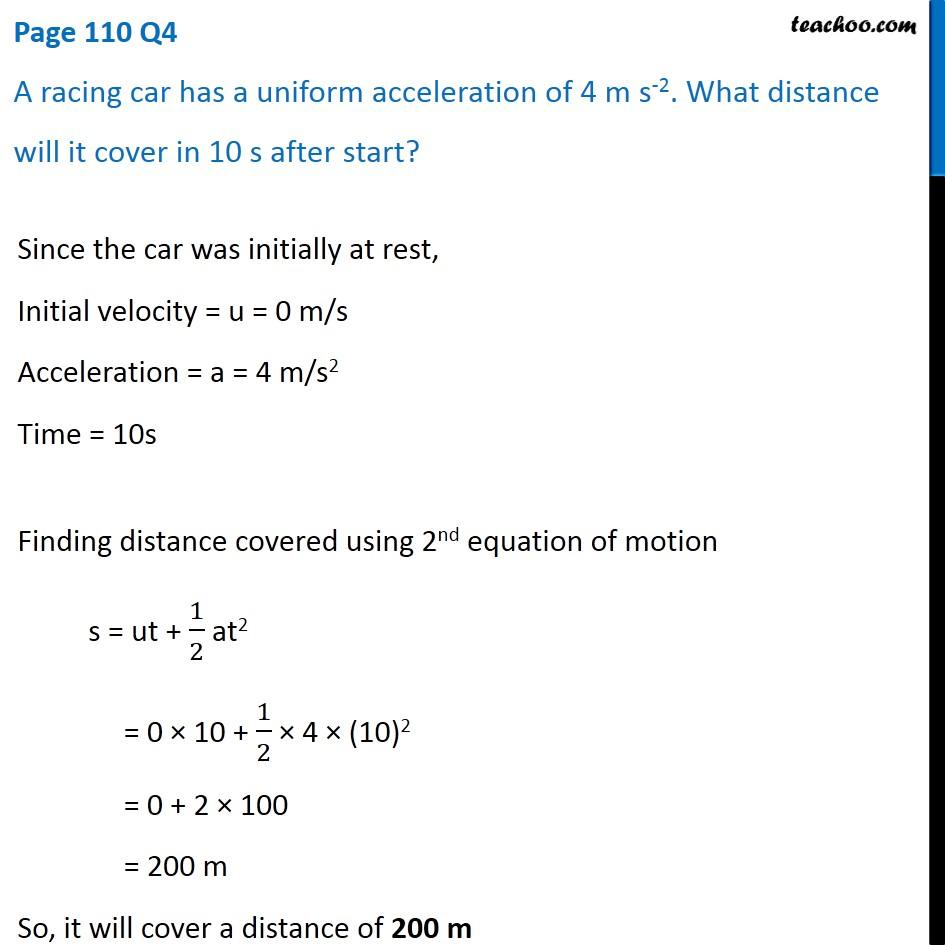Questions from Inside the chapter

Class 9
Chapter 8 Class 9 - MotionLearn in your speed, with individual attention - Teachoo Maths 1-on-1 Class

### Transcript

Page 110 Q4 A racing car has a uniform acceleration of 4 m s-2. What distance will it cover in 10 s after start? Since the car was initially at rest, Initial velocity = u = 0 m/s Acceleration = a = 4 m/s2 Time = 10s Finding distance covered using 2nd equation of motion s = ut + 1/2 at2 = 0 × 10 + 1/2 × 4 × (10)2 = 0 + 2 × 100 = 200 m So, it will cover a distance of 200 m